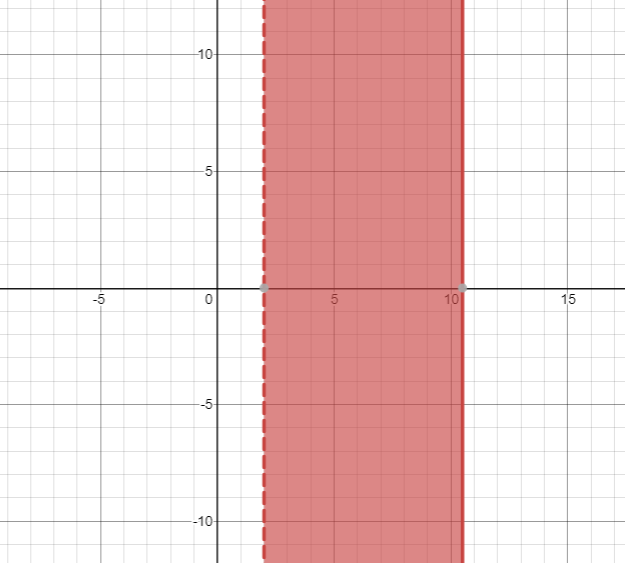# The solution of given linear inequality equation and plot a graph for it.### Precalculus: Mathematics for Calcu...

6th Edition
Stewart + 5 others
Publisher: Cengage Learning
ISBN: 9780840068071### Precalculus: Mathematics for Calcu...

6th Edition
Stewart + 5 others
Publisher: Cengage Learning
ISBN: 9780840068071

#### Solutions

Chapter 1.7, Problem 33E
To determine

## The solution of given linear inequality equation and plot a graph for it.

Expert Solution

2<x10.5

### Explanation of Solution

Given:

Linear inequality equation

16<2x131223

Calculation,

Linear inequality equation,

16<2x131223212<2x13128122<2x1382<2x8+132<2x212<x2122<x10.5

Now, graph of 16<2x131223Conclusion:

Hence, the values of x lies between the 2 and 10.5.

### Have a homework question?

Subscribe to bartleby learn! Ask subject matter experts 30 homework questions each month. Plus, you’ll have access to millions of step-by-step textbook answers!